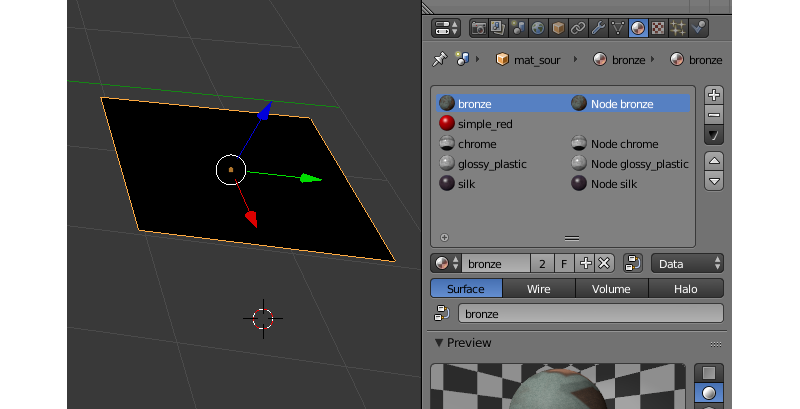# 材质¶

## 灯光参数¶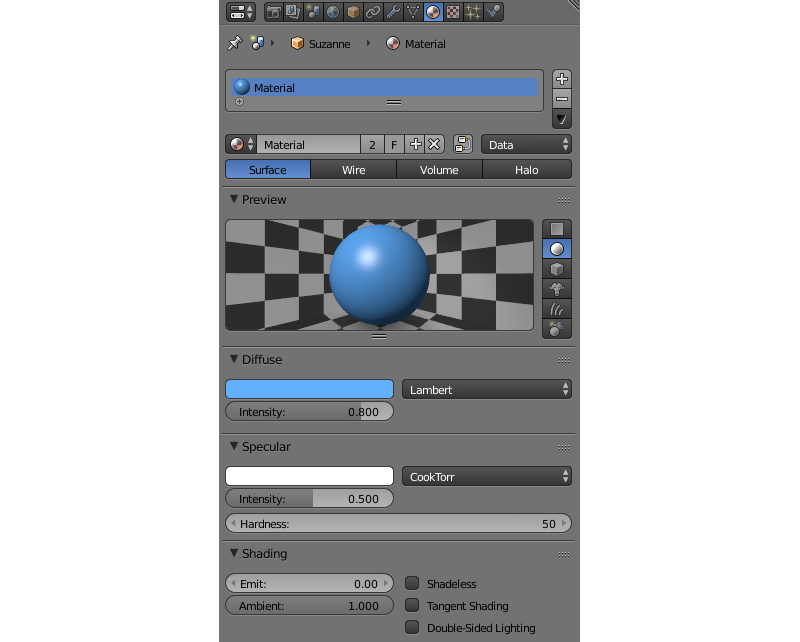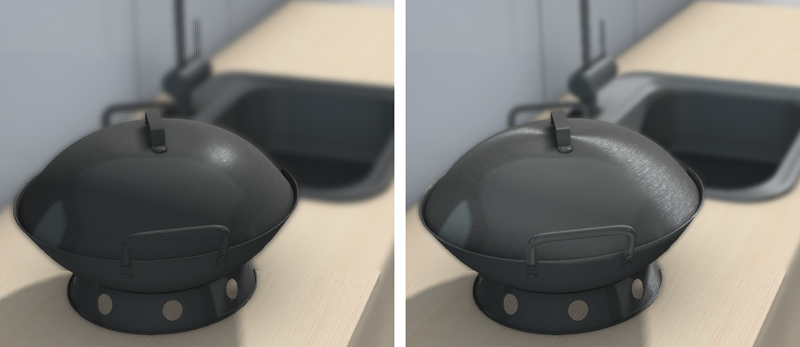## 透明¶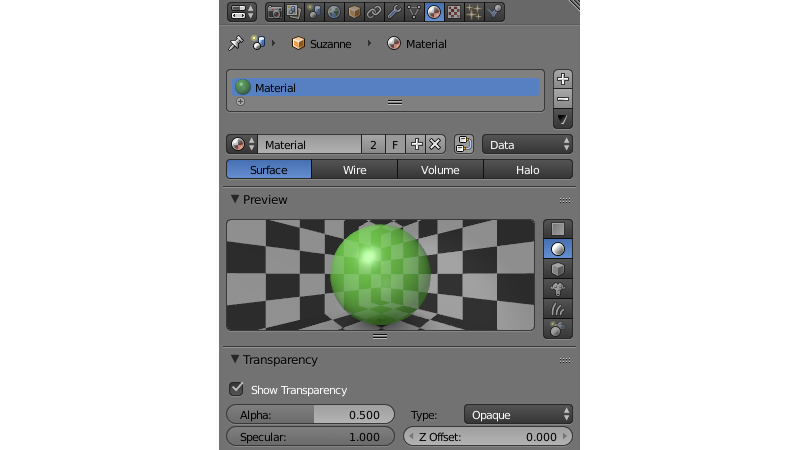### 类型¶

属性 > 材质 面板菜单中的 透明 ，可以选择透明度的类型。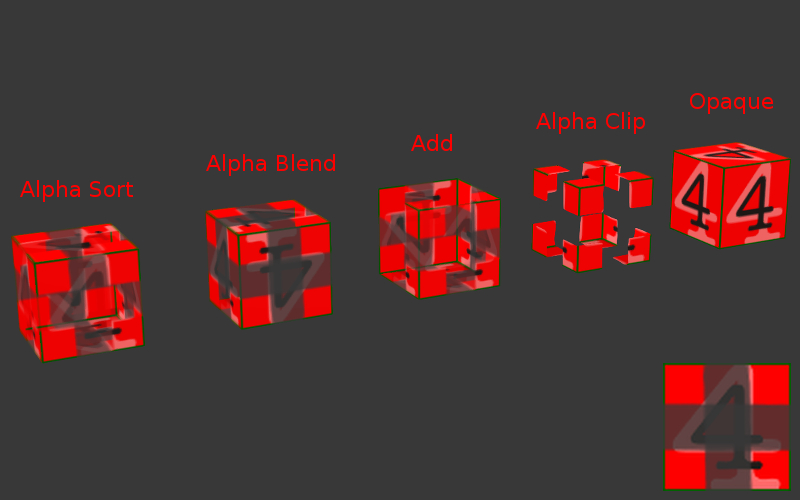Alpha 排序

Alpha抗锯齿

Alpha 混合

Alpha 剪切

## 反射¶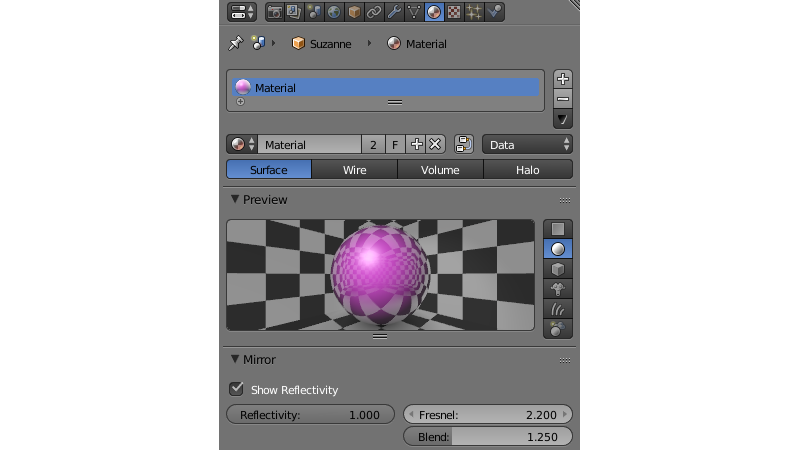材质 选项卡中的反射设置

### 动态反射¶

If you are using node materials, dynamic reflection will only work if an Extended Material node is present in the node tree.

#### 激活¶

1. 检查 Render > Reflections 和折射面板上的 Reflections 设置。

2. 反射 物体，启用 Object > Reflections 面板上的 Reflective 选项。

• 对平面的反射， Object > Reflections > Type 属性设置为 Plane。然后，添加一个空物体用作反射平面，例如通过执行 Add > Empty > Single Arrow。为方便起见重命名，在反射物体 Reflection plane 字段中指定其名称。

• 用方块贴图反射， Object > Reflections > Type 属性设置为 Cube.

3. 反射 物体如需要材质，设置 材质 > Mirror > Reflectivity 值。

• 在Blender中显示的物体上的反射需要设置 Mirror > Reflectivity > Show Reflectivity 。引擎将忽略此选项。

4. 反射性 物体，启用 Object > Reflections 面板上的 Reflexible 复选框。

### 菲涅耳反射效果¶

R = R0 + (1 − R0)(1 - cos θ)N, 在

R - 反射系数

R0 - 在成直角的表面中观看的右夹角下的反射系数 (即当 θ = 0)，

θ - 入射角 （即等于反射角度下的光进入相机），它由引擎实时计算，

N-指数。

#### 设置¶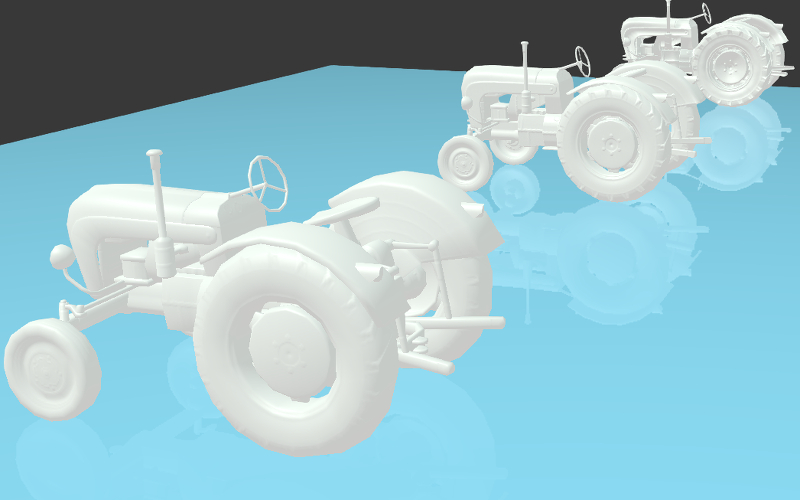## 渲染属性¶## 视图属性¶

Viewport 部分可以在 Blend4Web 面板上发现。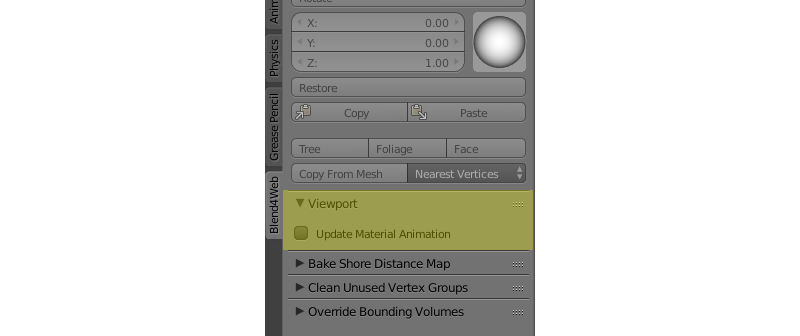## 引擎特定参数¶## 光晕材质¶

### 激活¶

Materials 选项卡下选择 光晕 类型 。建议也选择使用渐变的透明度类型 (Add, Alpha Blend 或者 Alpha Sort).## 材质API¶

API方法只能在添加到动态物体的材质上工作。

### 堆叠材质的方法¶

material API模块包括控制堆栈材质的每一个方面的方法。

var m_scenes    = require("scenes");
var m_material  = require("material");

...

var cube = m_scenes.get_object_by_name("Cube");

var diffuse_color = m_material.get_diffuse_color(cube, "MyMaterial");
var diffuse_color_factor = m_material.get_diffuse_color_factor(cube, "MyMaterial");
var diffuse_intensity = m_material.get_diffuse_intensity(cube, "MyMaterial");


var m_scenes    = require("scenes");
var m_material  = require("material");

...

var cube = m_scenes.get_object_by_name("Cube");

var specular_color = m_material.get_specular_color(cube, "MyMaterial");
var specular_color_factor = m_material.get_specular_color_factor(cube, "MyMaterial");
var specular_hardness = m_material.get_specular_hardness(cube, "MyMaterial");
var specular_intensity = m_material.get_specular_intensity(cube, "MyMaterial");


var m_scenes    = require("scenes");
var m_material  = require("material");

...

var cube = m_scenes.get_object_by_name("Cube");

var emit_factor = m_material.get_emit_factor(cube, "MyMaterial");
var alpha_factor = m_material.get_alpha_factor(cube, "MyMaterial");
var ambient_factor = m_material.get_ambient_factor(cube, "MyMaterial");

var extended_parameters = m_material.get_material_extended_params(cube, "MyMaterial");


var m_scenes    = require("scenes");
var m_material  = require("material");
var m_rgba      = require("rgba");

...

var cube = m_scenes.get_object_by_name("Cube");

m_material.set_diffuse_color(cube, "MyMaterial", m_rgba.from_values(1.0, 0.0, 0.0, 1.0));
m_material.set_diffuse_color_factor(cube, "MyMaterial", 0.05);
m_material.set_material_extended_params(cube, "MyMaterial", {fresnel: 0,
fresnel_factor: 1.25,
parallax_scale: 0,
parallax_steps: "5.0",
reflect_factor: 0});


### 节点材质的方法¶

• 到物体的链接，使用这种特殊的材质，

• 该材质的名称，

• 节点本身的名称

• 和包含该节点（如果存在的话）的节点组的名称。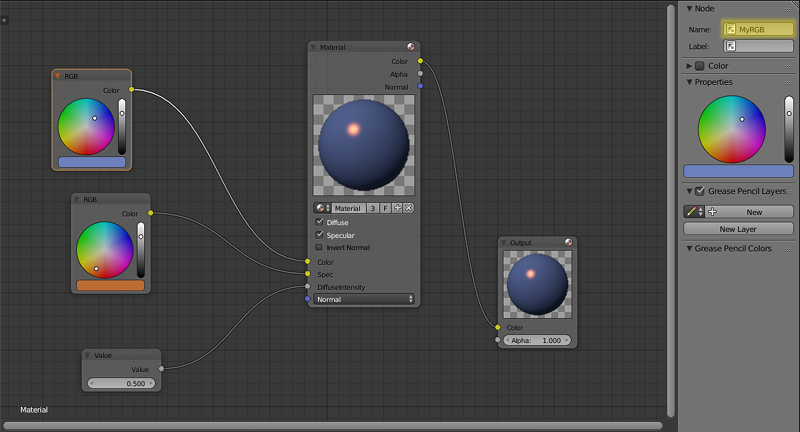var m_scenes    = require("scenes");
var m_material  = require("material");

...

var cube = m_scene.get_object_by_name("Cube");

var rgb_node_1 = m_material.get_nodemat_rgb(cube, ["MyMaterial", "MyRGB"]);
var rgb_node_2 = m_material.get_nodemat_rgb(cube, ["MyMaterial", "MyRGB_2"]);
var value_node = m_material.get_nodemat_value(cube, ["MyMaterial", "MyValue"]);


var m_scenes    = require("scenes");
var m_material  = require("material");

...

var cube = m_scene.get_object_by_name("Cube");

m_material.set_nodemat_value(cube, ["MyMaterial", "MyValue"], 0.8);
m_material.set_nodemat_rgb(cube, ["MyMaterial", "MyRGB"], 0.7, 0.9, 0.3);


var m_scenes    = require("scenes");
var m_material  = require("material");

...

var my_world = m_scene.get_world_by_name("World");

var world_rgb = m_material.get_nodemat_rgb(my_world, ["My_RGB_3"]);


### 替换纹理¶

API 方法还允许您替换纹理图像。这可以使用 textures 模块的 change_image() 方法：

var m_scenes  = require("scenes");
var m_tex = require("textures");

...

var my_cube = m_scenes.get_object_by_name("Cube");

m_tex.change_image(my_cube, "My_Texture", "./test.png");


## 材质继承¶

Blend4Web支持动态的材质切换。这个特性给用户一个机会可以配置模型外观非常对有用，诸如服装，一件家具，汽车或其他东西。继承材质特性可以在通过 change_texture 方法来调整堆叠材质和节点材质不够用的时候使用。

### 激活¶